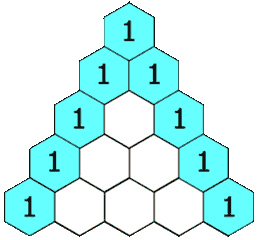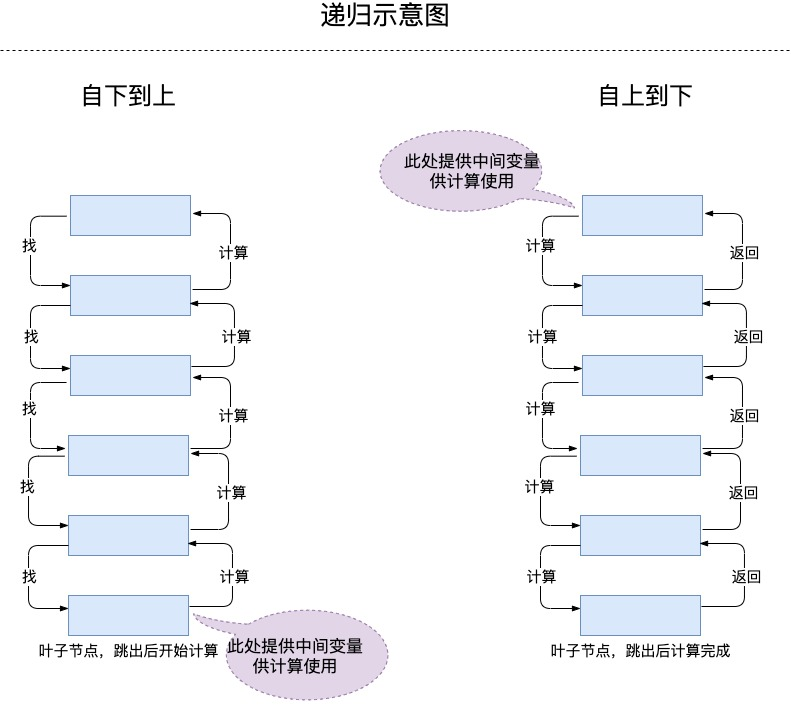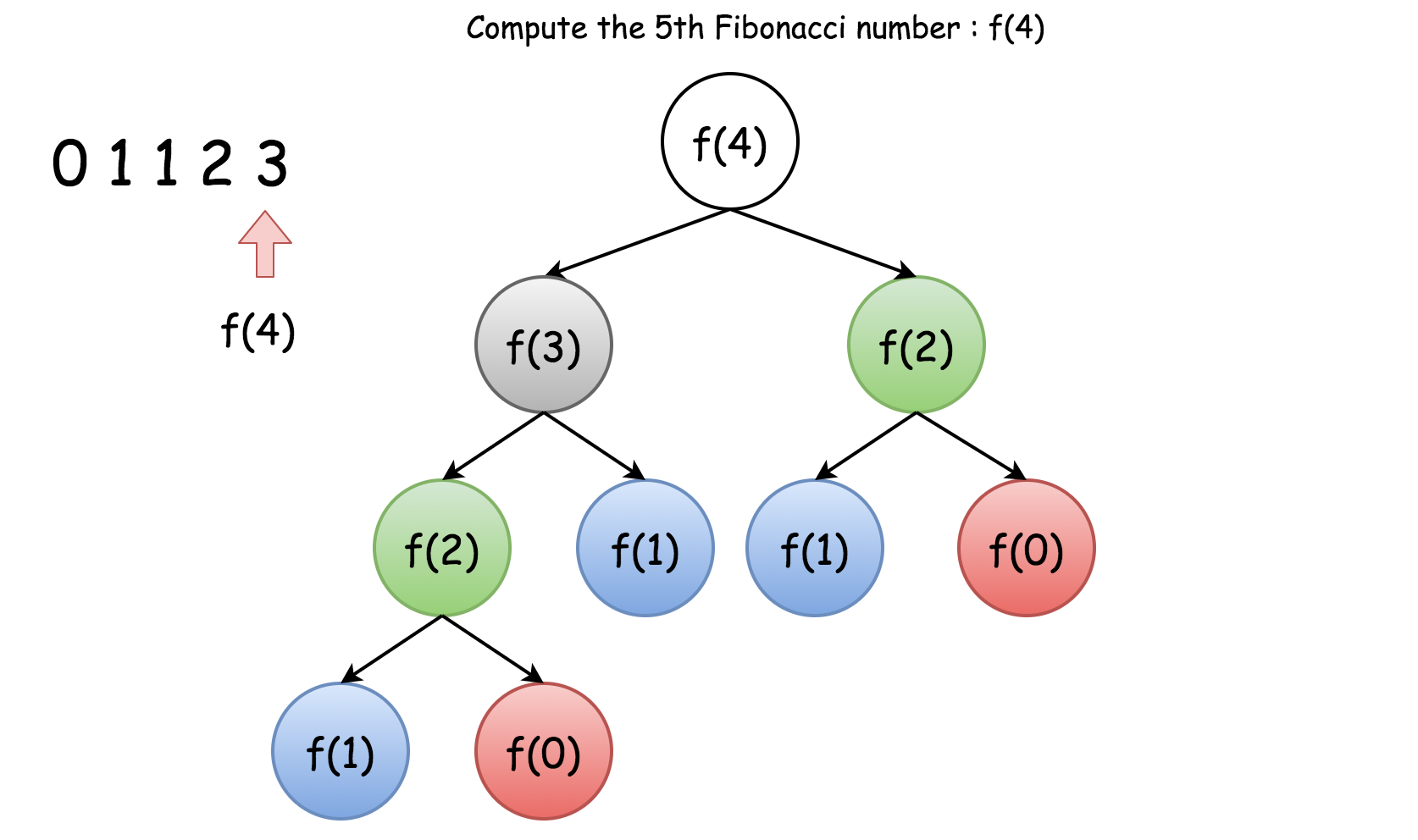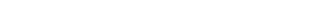## # 1. 递归的定义

• 递推关系：一个问题的结果与其子问题的结果之间的关系。
• 基本情况：不需要进一步的递归调用就可以直接计算答案的情况。可理解为递归跳出条件。

### # 1.1 递推关系• 递推关系：$f(i, j) = f(i - 1, j - 1) + f(i - 1, j)$
• 基本情况：$f(i,j) = 1$ ，当 $j=1$ 或者 $i=j$ 时。

## # 2. 如何写出一个递归函数

$1 + 2 + 3 + 4 + ... + n$ ，函数表达式为 $f(n) = f(n-1) + n$

### # 2.2 寻找递推关系（难点）

#### # 2.2.1 由下到上

/**
* 模拟程序执行过程：
* 5 + sum(4)
* 5 + (4 + sum(3)
* 5 + 4 + (3 + sum(2))
* 5 + 4 + 3 + (2 + sum(1))
* ------------------> 到达基本情况 sum(1) = 1 ，开始执行 ③ 行代码
* 5 + 4 + 3 + (2 + 1)
* 5 + 4 + (3 + 3)
* 5 + (4 + 6)
* (5 + 10)
* 15
* <p>
* 由下到上：最终从 1 + 2 + 3 + 4 + 5 计算...
* 递归函数「开始」部分调用自身，这个过程就是找到基本情况），然后根据返回值进行计算。
*/
public int sum(int n) {
if (n < 2) return n;       // ① 递归基本情况
int childSum = sum(n - 1); // ② 寻找基本情况
return n + childSum;       // ③ 根据返回值运算
}


• 寻找递推关系
• 寻找递归基本情况，跳出时返回基本情况的结果
• 修改递归函数的参数，递归调用
• 使用递归函数的返回值进行计算并返回最终结果
public 返回值 f(参数) {
if (基本情况条件) return 基本情况的结果;

修改参数；
返回值 = f(参数);

最终结果 = 根据参数与返回值计算
return 最终结果;
}


#### # 2.2.2 由上到下

/**
* 模拟程序执行过程：
* sum(5, 0)
* sum(4, 5)
* sum(3, 9)
* sum(2, 12)
* sum(1, 14)
* 15
* <p>
* 由上到下：最终从 5 + 4 + 3 + 2 + 1 计算...
* 递归函数「末尾」部分调用自身，根据逻辑先进行计算，然后把计算的中间变量传递调用函数。
* <p>
* 这种在函数末尾调用自身的递归函数叫做「尾递归」
*/
public int sum2(int n, int sum) {
if (n < 2) return 1 + sum;
sum += n;
return sum2(n - 1, sum);
}


• 寻找递推关系
• 创建新函数，将「由下到上-范式」中的最终结果计算依赖的中间变量提取为函数的参数
• 寻找递归基本情况，跳出时返回基本情况的结果与中间变量的计算结果（最终结果）
• 根据函数参数与中间变量重新计算出新的中间变量
• 修改参数
• 递归调用并返回（该处的返回由基本情况触发）
public 返回值 f(参数，中间变量) {
if (基本情况条件) return 基本情况的结果与中间变量的计算结果;

中间变量 = 根据参数与中间变量重新计算
修改参数；

return f(参数,中间变量);
}


#### # 2.2.3 由下到上、由上到下的区别### # 2.3 优化递归函数

• 充分分析基本情况（跳出条件），避免临界值跳不出递归，导致栈溢出。

• 分析递归深度，太深的递归容易导致栈溢出。

• 分析是否有重复计算问题，主要分析函数参数值是否会出现重复，直接代入递归的递推关系中运算即可。如果会出现重复使用数据结构记录。

比如：斐波那契数列 $f(n) = f(n-1) + f(n-2)$ ，如果直接采用该公式进行递归会重复计算很多表达式。• 分析数据溢出问题

• 将「由下到上」优化为「由上到下」，再改写为尾递归，再退化为循环结构。因为递归会对栈及中间变量的状态保存有额外的开销。

### # 2.4 改为循环

// Scala 编译前的尾递归写法，并注解为尾递归
@scala.annotation.tailrec
def sum2(n: Int, sum: Int): Int = {
if (n < 2) return sum + n
sum2(n - 1, sum + n)
}

// 编译后优化为循环结果
public int sum2(int n, int sum) {
while (true) {
if (n < 2) return sum + n;
sum += n;
n--;
}
}


// 并不是最后一行递归调用就是尾递归，下面例子其实是一个由下到上的递归写法，返回值与 n 有关。
def sum(n: Int): Int = {
if (n < 2) return n
return n + sum(n - 1)
}


## # 3. 案例实战-递归乘法

递归乘法。 写一个递归函数，不使用 * 运算符， 实现两个正整数的相乘。可以使用加号、减号、位移，但要吝啬一些。

输入：A = 1, B = 10
输出：10

输入：A = 3, B = 4
输出：12

public int multiply(int A, int B)


### # 3.1 审题思路

public int multiplyFor(int A, int B) {
int sum = 0;
while (A-- > 0) sum += B;
return sum;
}


### # 3.2 尝试递归

- 寻找递推关系
- 寻找递归基本情况，跳出时返回基本情况的结果 -> B
- 修改递归函数的参数，递归调用 -> multiply(A - 1, B) return sum
- 使用递归函数的返回值进行计算并返回最终结果 -> sum + B

public int multiply(int A, int B) {
if (A < 2) return B;          // 跳出时返回基本情况的结果
int sum = multiply(A - 1, B); // 先递归
return sum + B;               // 再计算，依赖递归的返回值
}


$f(a,b) = sum + f(a-n,b)$$sum$ 为已经计算了的 $n$$b$ 的和。

- 寻找递推关系
- 创建新函数，将「由下到上-范式」中的最终结果计算依赖的中间变量提取为函数的参数 -> multiply1Help 函数 sum 为中间变量
- 寻找递归基本情况，跳出时返回基本情况的结果与中间变量的计算结果（最终结果） -> return B + sum
- 根据函数参数与中间变量重新计算出新的中间变量 -> sum += B
- 修改参数 -> A - 1
- 递归调用并返回（该处的返回由基本情况条件触发）-> B + sum

public int multiply1(int A, int B) {
return multiply1Help(A, B, 0);
}

public int multiply1Help(int A, int B, int sum) {
if (A < 2) return B + sum;          // 跳出时返回基本情况的结果与中间变量的计算结果
sum += B;                           // 根据函数参数与中间变量重新计算出新的中间变量
return multiply1Help(A - 1, B, sum);// 由基本情况条件触发决定，最终返回 B + sum
}


### # 3.3 尝试优化

• 如果 A > B ，处理 A 次，因此考虑使用 $M=MIN(A,B)$ 作为循环次数。比如 $100 * 1$ 时可优化 100 倍
• 分析重复计算问题，目前没有。
• 分析参数的边界问题，目前没有。题目给定约束都是正整数。
• 优化时间复杂度，重新分析递推关系为 $a * b = (a/2) * (b*2)$，直到 $a/2$ 等于 $1$ 时，$b$ 就是最终结果，我们基于二进制的位移操作进行优化， 但是考虑如果是奇数除以 $2$ 时会丢失一个 $b$，这样复杂度优化为 $O(\log_2n)$
优化过程示例：a * b = (a/2) * (b*2)

8 * 9
4 * 18
2 * 36
1 * 72
72

7 * 9
9 + (3 * 18)        -> 7/2 时丢失一个 9
9 + 18 + (1 * 36)   -> 3/2 时丢失一个 18
9 + 18 + 36
63


public int multiply2(int A, int B) {
return (A < B) ? multiply2Help(A, B, 0) : multiply2Help(B, A, 0); // 寻找最小循序次数
}

// missPart 为奇数除以 2 时丢失的部分
public int multiply2Help(int A, int B, int missPart) {
if (A < 2) return missPart + B;   // 最终结果 = 丢失的部分 + 最终 B 的结果
missPart += (A & 1) == 1 ? B : 0; // 是否为奇数，奇数时记录丢失的部分
return multiply2Help(A >> 1, B << 1, missPart); // 位移运算优化
}


## # 4. 案例实战-青蛙跳台阶

1. 寻找基本情况：剩余一个台阶时只能有一种跳法 $f(1) = 1$，剩余两个台阶时只能有两种跳法 $f(2) = 2$，或者剩余 3 个台阶时有 3 种跳法（1-1-1、1-2、2-1）

2. 寻找递推关系：如果跳 1 级台阶就少一个，结果为 $f(n-1)$ 种，如果跳 2 级台阶就少 2 个，结果为 $f(n-2)$ 种。
所以推导递推关系为 $f(n) = f(n-1) + f(n-2)$

3. 优化递归：考虑重复计算问题，因为涉及减法运算，肯定会出现重复代入 $f(n)$ 计算，因此考虑使用数据结构保存计算过的结果。分析数据溢出问题，如果没有给定约束条件，要考虑返回跳法是否会溢出。

/**
* - 寻找递推关系 f(n)=f(n-1)+f(n-2)
* - 寻找递归基本情况，跳出时返回基本情况的结果 f(1) = 1,f(2) = 2
* - 修改递归函数的参数，递归调用 -> 套入递推关系，当前 n 台阶跳法为 count=f(n-1)+f(n-2)
* - 使用递归函数的返回值进行计算并返回最终结果 -> 递归返回跳法数 count 即为最终结果
*/
public int numWays1(int n) {
if (n == 1) return 1;
if (n == 2) return 2;
int count = numWays1(n - 1) + numWays1(n - 2);
return count;
}

// 优化思路，2 个 if 可有优化 为 if (n <= 2) return n; 减少执行次数
// 递归函数重复计算问题，使用临时变量保存

private final Map<Integer, Integer> statusRecord = new HashMap<>();

public int numWays(int n) {
if (n <= 2) return n; // if 判断比计算状态判断开销小，因此先进行 if
final Integer integer = statusRecord.get(n); // 计算状态判断，已经计算直接返回
if (integer != null) return integer;
int count = numWays(n - 1) + numWays(n - 2);
statusRecord.put(n, count); // 计算的结果保存至状态表
return count;
}

// 至此除了数据溢出问题没有处理，重复计算已优化。


/**
* 由上到下的范式套入实现：
*
* - 寻找递推关系
* 由下到上递推关系为，f(n) = f(n-1) + f(n-2) 相当于从 n-1 的计算过程，先从 n 找到 1，然后在从 1 累加到 n 的过程
* 我们改为从 1-n 的过程，f(i+1) = f(i) + f(i-1) ， i+1==n 时计算结束，累加的过程变量需要我们提取为中间变量参数
*
* - 创建新函数，将「由下到上-范式」中的最终结果计算依赖的中间变量提取为函数的参数
* 将 f(i)，f(i-1) 的变量保存，初始调用我们使用 f(2) = f(1) + f(0) = 1 + 1 作为初始状态
*
* - 寻找基本情况，跳出时返回基本情况的结果与中间变量的计算结果（最终结果）-> if (i >= n) return a + b;
*
* - 根据函数参数与中间变量重新计算出新的中间变量
* f(i) = f(i-1) + f(i-2) = a + b
* f(i+1) = f(i) + f(i-1) = (a+b) + b
*
* - 修改参数 -> i + 1 递进一步
*
* - 递归调用并返回（该处的返回由基本情况条件触发）
*/
public int numWaysTail(int n) {
if (n < 2) return n;
return numWaysTailHelp(n, 2, 1, 1);
}

private int numWaysTailHelp(int n, int i, int a, int b) {
if (i >= n) return a + b;
return numWaysTailHelp(n, i + 1, a + b, a);
}

// 因为是从 1-n 的计算，所以不会出现重复计算过程。


public int numWaysFor(int n) {
if (n < 2) return n;

int i = 2; int a = 1; int b = 1; // 与尾递归 numWaysTailHelp 一致
int count = a + b; // 保存次数，将尾递归的返回值提取为变量

while (i <= n) { // 1-n 的过程
// 因为 f(i) = f(i-1) + f(i-2) = a + b
// 下次迭代时 f(i+1) = f(i) + f(i-1) = (a+b) + b
count = a + b;
b = a;
a = count;
i++;
}
return count;
}


## # 总结

• 你要写哪种类型的递归，从上算还是从下算，这决定了你如何确认递推关系
• 分析基本情况
• 寻找递推关系，在递推关系中提取中间变量
• 套入上文中的递归范式
• 按上文中的优化点进行优化

## # 参考